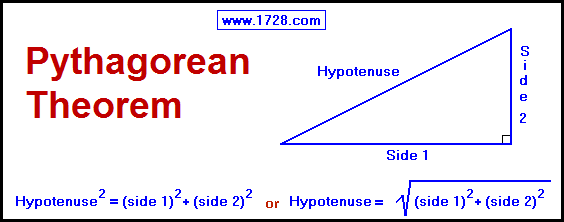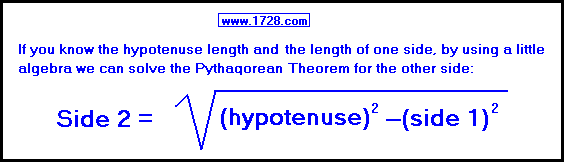Pythagorean Theorem CalculatorThe Pythagorean Theorem is used for calculating the hypotenuse length of a right triangle. A right triangle with sides 6 and 8 will have a hypotenuse length of 10 because: Hypotenuse = Square Root Of ( 6² + 8²) Hypotenuse = Square Root Of ( 36 + 64) Hypotenuse = Square Root Of ( 100) Hypotenuse = 10EXAMPLE: One side of a right triangle measures 5 and the hypotenuse equals 13. What is the length of the other side? Side 2 = Square Root of (13 ² - 5 ²) Side 2 = Square Root of (169 - 25) Side 2 = Square Root of (144) Side 2 = 12 As an added bonus, all 3 angles and the triangle area will automatically be calculated.
Do you want to solve for:

or
>>>>>
>>>>>

>>>>>